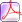#### Algebra 2 WorksheetsAlgera pack of worksheets for 6th grade students on algebra 2.

### Consumer Math pdfsLearn 6th grade consumer math and money - dime, nickle, quater, dollars and more

### Decimals worksheetsMath exercises for grade 6 on decimals, addition, subtraction, algebra and more.

### Fractions worksheetsFractions addition, subtraction, division, multiplication, word problems and division.

### Geometry worksheetsSolve several problems related to geometry with shapes like rectangle, circumference, complex figures etc.

### Inequalities WorksheetsAlgebra worksheets and printable tests for sixth graders to learn.

### Integers Printables### Measurements sheetsLearn measurements and metric systems through the collection of worksheets on this page.

### Number SequencesLearn number sequences like arithmetic and geometric progressions of a sequence of numbers.

### Tables & Graphs sheetsWorksheets featuring questions on tables, data and graphical representations.

### Percentages & RatiosSolve varied word and number problems related to 6th grade ratio.

### Subtraction worksheetsLearn how to subtract large numbers up to million pdf

### Pre-algebra worksheetsSolve linear equations, finding missing variables, algebraic expressions and more.

### Probability & StatsFind probabilities and possible outcomes by solving problems on this test papers.

### Pythagorean TheoremIn search for sixth grade math test papers with answer keys, come here.

### Ratios & ProportionsLearn about ratios, proportions, converstions and more through these tests papers.

# MATH WORKSHEETS FOR SIXTH 6TH GRADE - PDF

Math worksheets for sixth grade children covers all topics of 6th grade such as Graphs, Data, Fractions, Tables, Subtractions, Pythagoras theorem, Algebra, LCM, HCF, Addition, Round up numbers , Find 'X' in addition equations, Metric systems, Coordinate geometry, Surface Areas, Order of operations, Decimals, Probability, Money & more. This collection will unlock a wealth of math resources which teachers can use to train their kids in grade 6. Each sheet has an answer key attached on the second page making it easy for parents and teachers. 6th grade math worksheets free | 6th grade math worksheets common core | 6th grade math worksheets free printable.

###Rounding up Numbers Math WorsheetsAlgebra is fun. These games will help kids practice algebra in a fun way. Kids relate very well to games . Several algebra topics are covered in the form of interactive games and include the following:

Algebra Worksheets & PrintableThese worksheets are printable PDF exercises of the highest quality. Writing reinforces Maths learnt. These math worksheets for children contain pre-algebra & Algebra exercises suitable for preschool, kindergarten, first grade to eight graders, free PDF worksheets, 6th grade math worksheets . The following algebra topics are covered among others:This section contains worked examples of math problems and vital algebra formulae that are needed through out the theme. It is very important to master using these formulae though some times presenting them in the most simplified manner is necessary for young learners. This section is a step-by-step presentation of how to use algebra formulae on all the topics covered in this site which include formulae on -linear equations, inequalities, decimals, fractions, exponents, graphing linear equations, binomial theorem, pythagoras theorem, quadratic equations, algebraic expressions, factorization, ratios, geometry, integers, order operations, angles, simple equations, slope, arithmetic progression, LCM & HCF, coefficients, quadratic equations, square roots & more-

These math quizzes range from multiple choice algebra quizzes, gap fill quizzes, matching exercises, hotspot quizzes with graphics and more for interactive algebra & pre-algebra practice. - for first grade, second grade, third grade, fourth grade, fifth grade sixth grade and eight grade.

Web site General Content:

This math site is all about the following: Algebra exercises for children, Algebra & pre - algebra for kids, children, Algebra activities Online for children, Algebra games for kids, elementary algebra, first grade algebra, algebra games, worksheets, printable, exercises, free online exercises, free algebra downloads, printable PDF,linear equations, inequalities, decimals, fractions, exponents, graphing linear equations, binomial theorem, pythagoras theorem, quadratic equations, algebraic expressions, factorization, ratios, geometry, integers, order operations, angles, simple equations, slope, arithmetic progression, LCM & HCF, coefficients quadratic equations, square roots & more-

math, printable Algebra exercises, Algebra worksheets, Pre -algebra worksheets, pre-algebra, , Algebra for children, Algebra topics, Algebraic processes, algebra for kids, worksheets, printable on algebra, linear equations, polynomials, introduction to algebra, pythagoras theorem, fractions, algebra online, algebra is fun, algebra printable games, algebra,cool maths, Fun brain, formulae & more